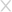• 手机版微信扫一扫

• 投稿

## CoolShell解密游戏的WriteUp

2014-08-18 331109人围观 ，发现 11 个不明物体

```#include <stdio.h>
int  p, r, q;
char a, f, b, o, *s=f;
void interpret(char *c)
{
char *d;
r++;
while( *c ) {
switch(o=1,*c++) {
case '<': p--;        break;             case '>': p++;        break;
case '+': a[p]++;     break;
case '-': a[p]--;     break;
case '.': putchar(a[p]); fflush(stdout); break;
case ',': a[p]=getchar();fflush(stdout); break;
case '[':
for( b=1,d=c; b && *c; c++ )
b+=*c=='[', b-=*c==']';
if(!b) {
c[-1]=0;
while( a[p] )
interpret(d);
c[-1]=']';
break;
}
case ']':
puts("UNBALANCED BRACKETS"), exit(0);
case '#':
if(q>2)
printf("- - - - - - - - - -/n%*s/n",*a,a,a,a,a,a,a,a,a,a,3*p+2,"^");
break;
default: o=0;
}
if( p100)
puts("RANGE ERROR"), exit(0);
}
r--;
}
main(int argc,char *argv[])
{
FILE *z;
q=argc;
if(z=fopen(argv,"r")) {
while( (b=getc(z)) > 0 )
*s++=b;
*s=0;
interpret(f);
}
}```

`gcc test.c -o test`

`<<<<<<<<<<<>>>>>>>>>>>>>>>----.++++<<<<<<<<<<<<<<<>>>>>>>>>>>>>>---.+++<<<<<<<<<<<<<<>>>>>>>>>>>>>>----.++++<<<<<<<<<<<<<<.`

`./test test.bf`1-Multiply

`2,3,6,18,108,?`

`What is the meaning of life, the universe and everything?`

```根据道格拉斯·亚当斯的小说《银河系漫游指南》，42是“生命、宇宙以及任何事情的终极答案”。因此，42也经常被用来向这一作品致敬，例如：

2-Keyboard```//这是给出的代码
macb() ? lpcbyu(&gbcq/_21%ocq12_=w(gbcq)/_dak._=}_ugb_[0q60)s+```
```//这是QWERTY布局下代码
main() { printf(&unix["21%six12"],(unix)["have"]+"fun"-0x60);}```3-QR Code`[abcdefghijklmnopqrstuvwxyz] <=> [pvwdgazxubqfsnrhocitlkeymj]`

```#Thanks For Code by Mutalisk
content_de = '''Wxgcg txgcg ui p ixgff, txgcg ui p epm. I gyhgwt mrl lig txg ixgff wrsspnd tr irfkg txui \
hcrvfgs, nre, hfgpig tcm liunz txg crt13 ra "ixgff" tr gntgc ngyt fgkgf.'''.lower()

content = ''

keychr_de = 'pvwdgazxubqfsnrhocitlkeymj'
keychr = 'abcdefghijklmnopqrstuvwxyz'

for s_chr in content_de:
if s_chr in keychr_de:
content = content + keychr[keychr_de.index(s_chr)]
else:
content = content + s_chr

print content````Where there is a shell, there is a way. I expect you use the shell command to solve this problem, now, please try using the rot13 of “shell” to enter next level.`

`只要有shell就会有办法咩我希望你捏，能使用shell命令去解决这个问题咩，那么现在捏，请使用rot13来过关咩`

`You really are close, but the “shell” is not the final answer.`

`你已经很接近正确答案了咩，但可惜shell不是最终的答案咩.`

`echo &#039;shell&#039;|tr &#039;[A-Za-z]&#039; &#039;[N-ZA-Mn-za-m]&#039;`

4-cat`([A-Z])([0-9])[a-z](\2)(\1)|([0-9])([A-Z])[a-z](\6)(\5)````共找到 9 处匹配：
E1v1E
4FaF4
9XrX9
O3i3O
0MaM0
4GbG4
M5l5M
0WeW0
Y0s0Y```

5-variables

```dim a
function main
start = "2014"
a = GetHTTP("http://fun.coolshell.cn/n/"&start)
Print(a)
for ForCount = 1 to 999999
CStr(a)
a = GetHTTP("http://fun.coolshell.cn/n/"& a )
Print(a)
next
end function```6-treez, W, b, T, g, h, H, V, 3, o, P, p, 8, L, F, G, u, A, f, n, 0, 1, r, m, w, x, J, 7, e, i, Q, Y, Z, 2, 5, v, K, q, k, 9, y, C, B, N, D, U, 4, l, c, M, I, E, a, j, 6, S, R, o, X, s, d

`echo U2FsdGVkX1+gxunKbemS2193vhGGQ1Y8pc5gPegMAcg=|openssl enc -aes-128-cbc -a -d -pass pass:zWp8LGn01wxJ7`7-N Queen

```/**
* n皇后问题
* date       : 2010-3-12
* author     : lee
* change     ：LinE
* change date：2014-8-4
*/

#include <stdio.h>
#include <stdlib.h>
#include <math.h>

#define QUEEN 9    // the number of the queen
#define INITIAL -10000  //defines the initial value of  the board

//container
int a[QUEEN];

//check if the queen can be placed on the position
int valid(int row, int col);
//initialize the board
void clear();
//print the result
void print();
//run the n-queen program
void queen();

int main(void)
{
clear();
queen();
return 0;
}

void clear()
{
int *p;
for (p = a; p < a + QUEEN; ++p) {
*p = INITIAL;
}
}

void print()
{
int i,j,l;
for (i = 0; i < QUEEN; ++i) {
for (j = 0; j < QUEEN; ++j) {
if (a[i] != j) {
//printf("%c ", &#039;.&#039;);
}
else
{
l=j+1;
printf("%d",l);
//printf("%c ", &#039;#&#039;);
}
}
}
printf("\n");
//printf("--------------------------------------------\n");
}

int valid(int row, int col)
{
int i;
for (i = 0; i < QUEEN; ++i) {
if (a[i] == col || abs(i - row) == abs(a[i] - col))
return 0;
}

return 1;
}

void queen()
{
int n = 0;
int i = 0, j = 0;
while (i < QUEEN) {

while (j < QUEEN) {
if (valid(i, j)) { //test if the queen can be placed on the position
a[i] = j;     //place the queen on the next line
j = 0;
break;
} else {         // if not, check the next position
++j;
}
}

if (a[i] == INITIAL) {   //if the current queen can&#039;t find its place
if (i == 0)        // and this is the first line ,then program end
break;
else  {           //else   backtrack
--i;
j = a[i] + 1;
a[i] = INITIAL;
continue;
}
}

if (i == QUEEN - 1) {  //already got a solution, print the result
print();
//  _sleep(600);
j = a[i] + 1;
a[i] = INITIAL;
continue;

}

++i;      // go on to place the queen on the next line if has any more
}
}``````gcc queen.c -o queen
./queen>code.txt```

```<?php
\$f = fopen("/root/Desktop/test/code.txt","r");
\$passwd="zWp8LGn01wxJ7";
\$hash="e48d316ed573d3273931e19f9ac9f9e6039a4242";
while (!feof(\$f))
{
\$code = fgets(\$f);
if (sha1(\$passwd.\$code)===\$hash) {
//因为fgets自带了一个换行符，故这里不需要再添加"\n"了
echo \$code;
}
}
fclose(\$f)
?>```8-Excel Column

C—-3

O—-15

L—-12

S—-19

H—-8

E—-5

```COOLSHELL = 3*26^8+15*26^7+15*26^6+12*26^5+19*26^4+8*26^3+5*26^2+12*26^1+12*26^0 = 751743486376
SHELL = 19*26^4+8*26^3+5*26^2+12*26^1+12*26^0 = 8826856```COOLSHELL / SHELL = 85165

```//个位计算
85165 / 26 = 3275
85165 % 26 = 15   //O
//十位计算
3275 / 26 = 125
3275 % 26 = 25   //Y
//百位计算
125 / 26 = 4
125 % 26 = 21   //U
//千位计算
4 / 26 = 0
4 % 26 = 4    //D```

```import math

COOLSHELL = 3*26**8+15*26**7+15*26**6+12*26**5+19*26**4+8*26**3+5*26**2+12*26**1+12*26**0
SHELL = 19*26**4+8*26**3+5*26**2+15*26**1+15*26**0
print &#039;COOLSHELL=&#039;,COOLSHELL
print &#039;SHELL=&#039;,SHELL
NUM = int(COOLSHELL / SHELL) + 1
print &#039;COOLSHELL / SHELL=&#039;,NUM
temp = NUM
a = temp / 26
b = temp % 26
c4 = chr( b + 64)
print &#039;a=&#039;,a
print &#039;b=&#039;,b
print c4
temp = a
a = temp / 26
b = temp % 26
c3 = chr( b + 64)
print &#039;a=&#039;,a
print &#039;b=&#039;,b
print c3
temp = a
a = temp / 26
b = temp % 26
c2 = chr( b + 64)
print &#039;a=&#039;,a
print &#039;b=&#039;,b
print c2
temp = a
a = temp / 26
b = temp % 26
c1 = chr( b + 64)
print &#039;a=&#039;,a
print &#039;b=&#039;,b
print c1
print &#039;Pass is :&#039;,c1+c2+c3+c4```

9-Fraternal Organisation```其它
1）本次活动中，有一个隐藏关卡，还没有人找出来。要能达到隐藏关卡，需要完成所有的题目。
2）活动的通关页是HelloWorld，这意味着——这仅仅是个开始。shutdown.rar，哈，果断后缀改名为.rar，然后发现打开以后有个名为helloworld的文本文档[原作者博客地址]### 这些评论亮了

• (1级) 回复
我用了webrobots....
)13(

### 已有 11 条评论

• (4级)  2014-08-18 回复 1楼

乌云知识库8.5号就发出来了。。。

• (6级)  2014-08-18 回复

@千秋丶千年  貌似木有隐藏关卡 前天晚上才看见原作者添加了隐藏关卡

• (1级)  2014-08-20

@pynwolf  隐藏关卡早就有了，我一开始做完之后没发现，到第二天题目作者公布还曾记得时候注明过，然后才发现的····

• (6级)  2014-08-22

@yw9381  然后我正看你博客的过程中 发现你更新了 …………

• (1级)  2014-08-24

@pynwolf  后面又更新了一些，主要是正则部分，写了一些自己的理解···

• (1级)  2014-08-18 回复 2楼

我用了webrobots….

•fake  2014-08-19 回复 3楼

看起来不错 ctf比赛和真实入侵真的两码事

• (1级)  2014-08-20 回复 4楼

貌似这文章是我写的 – -！乌云里也是我自己发的，在ParSec也发了一份，毕竟是一份子嘛

• (1级)  2014-08-20 回复 5楼

第一题p100应该是p>100吧

• (1级)  2014-08-21 回复

@haodongxu  恩，WordPress吧那个>吃掉了····

•刘德华  2014-09-20 回复 6楼### 活动预告### 填写个人信息

• 可以给我们打个分吗？